# Logic

A man can drink a barrel of water for 26 days, woman for 48 days. How many days will a barrel last between them?

Result

x =  16.865 d

#### Solution:

x*(1/26 + 1/48) = 1

37x = 624

x = 62437 ≈ 16.864865

Calculated by our simple equation calculator.

Leave us a comment of example and its solution (i.e. if it is still somewhat unclear...):Math student
Very poor English! Who phrased this problem(?) as it is not stated correctly - it is incoherent!

2 months ago  2 LikesMath student
Extremely poor wording! A man drinks a barrel of water for 26 days, woman for 48 days, for how many days they drink barrel together? Who wrote this???? It should be, a man can drink...., and a woman...., how many days will a barrel last between them (or for both of them). Who ever wrote this question has very poor English skills and should not be writing math word problems using English!

2 months ago  1 Like#### To solve this example are needed these knowledge from mathematics:

Looking for help with calculating harmonic mean? Looking for a statistical calculator? Need help calculate sum, simplify or multiply fractions? Try our fraction calculator. Do you have a linear equation or system of equations and looking for its solution? Or do you have quadratic equation?

## Next similar examples:

1. PumpWhat power has a pump output to move 4853 hl of water to a height of 31 m for 8 hours?
2. Forestry workersIn the forest is employed 56 laborers planting trees in nurseries. For 8 hour work day would end job in 37 days. After 16 days, 9 laborers go forth? How many days are needed to complete planting trees in nurseries by others, if they will work 10 hours a d
3. Weeks38 weeks is equal to how many days?
4. Christmas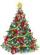Calculate how much of the school year (202 days long) take Christmas holidays 19 days long. Expressed as a decimal number and as a percentage.
5. HandsThe clock shows 12 hours. After how many minutes will agle between hour and minute hand 90°? Consider the continuous movement of both hands hours.
6. Motion problemFrom Levíc to Košíc go car at speed 81 km/h. From Košíc to Levíc go another car at speed 69 km/h. How many minutes before the meeting will be cars 27 km away?
7. Cyclist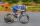A cyclist passes 88 km in 4 hours. How many kilometers he pass in 8 hours?
8. ServerCalculate how many average minutes a year is the web server is unavailable, the availability is 99.99%.
9. Holidays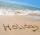Calculate how many hours take holidays, if take 5 weeks.
10. MovementFrom the crossing of two perpendicular roads started two cyclists (each at different road). One runs at average speed 28 km/h, the second at average speed 24 km/h. Determine the distance between them after 45 minutes cycling.
11. MotionIf you go at speed 3.7 km/h, you come to the station 42 minutes after leaving train. If you go by bike to the station at speed 27 km/h, you come to the station 56 minutes before its departure. How far is the train station?
12. Bus vs. trainBus started from point A 10 minutes before the train started from the same place. The bus went an average speed of 49 km/h, train 77 km/h. To point B train and bus arrived simultaneously. Calculate time of train journey, if train and bus travelled the.
13. ŽSRCalculate fixed annual personnel costs of operating monorail line 118 km long if every 5 km is station, which serve three people - one dispatcher and two switchman in 4-shift operation. Consider the average salary of the employee 885 €.
14. TimeWrite time in minutes rounded to one decimal place: 5 h 28 m 26 s.
15. Daily average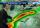Calculate the average temperature during the day, when 13 hours was 22 °C and 11 hours was 17 °C.
16. The cyclist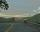The cyclist went from village to town. First half of journey went at 20 km/h. The second half of the journey, which mostly fell, went at 39 km/h. All journey took 88 minutes. Calculate the distance from the village to the town.
17. Negative percentage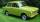In 2004, the company had a loss of 40900 Euros. Two years later he was already in profit 48900 Eur. Calculate what percentage of the company increased profits in this two years.